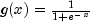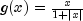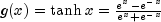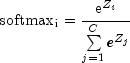JMSLTM Numerical Library 7.2.0
com.imsl.datamining.neural

## Interface Activation

• All Superinterfaces:
Serializable

```public interface Activation
extends Serializable```
Interface implemented by perceptron activation functions.

Standard activation functions are defined as static members of this interface. New activation functions can be defined by implementing a method, `g(double x)`, returning the value and a method, ``` derivative(double x, double y)```, returning the derivative of ```g ``` evaluated at x where y = g(x).

Feed Forward Class Example 1, `Perceptron`
• ### Field Summary

Fields
Modifier and Type Field and Description
`static Activation` `LINEAR`
The identity activation function, g(x) = x.
`static Activation` `LOGISTIC`
The logistic activation function,.
`static Activation` `LOGISTIC_TABLE`
The logistic activation function computed using a table.
`static Activation` `SOFTMAX`
The softmax activation function.
`static Activation` `SQUASH`
The squash activation function,`static Activation` `TANH`
The hyperbolic tangent activation function,.
• ### Method Summary

Methods
Modifier and Type Method and Description
`double` ```derivative(double x, double y)```
Returns the value of the derivative of the activation function.
`double` `g(double x)`
Returns the value of the activation function.
• ### Field Detail

• #### LINEAR

`static final Activation LINEAR`
The identity activation function, g(x) = x.
• #### LOGISTIC

`static final Activation LOGISTIC`
The logistic activation function,.
• #### LOGISTIC_TABLE

`static final Activation LOGISTIC_TABLE`
The logistic activation function computed using a table. This is an approximation to the logistic function that is faster to compute.

This version of the logistic function differs from the exact version by at most 4.0e-9.

Networks trained using this activation should not use ``` Activation.LOGISTIC``` for forecasting. Forecasting should be done using the specific function supplied during training.

• #### SOFTMAX

`static final Activation SOFTMAX`
The softmax activation function..
• #### SQUASH

`static final Activation SQUASH`
The squash activation function,• #### TANH

`static final Activation TANH`
The hyperbolic tangent activation function,.
• ### Method Detail

• #### derivative

```double derivative(double x,
double y)```
Returns the value of the derivative of the activation function.
Parameters:
`x` - A `double` which specifies the point at which the activation function is to be evaluated.
`y` - A `double` which specifies `y = g(x)`, the value of the activation function at `x`. This parameter is not mathematically required, but can sometimes be used to more quickly compute the derivative.
Returns:
A `double` containing the value of the derivative of the activation function at `x`.
• #### g

`double g(double x)`
Returns the value of the activation function.
Parameters:
`x` - A `double` is the point at which the activation function is to be evaluated.
Returns:
A `double` containing the value of the activation function at `x`.
JMSLTM Numerical Library 7.2.0